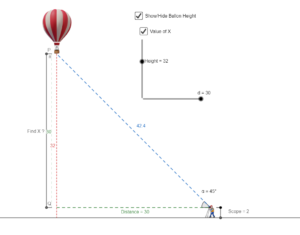# Real-Life Example of Trigonometry

A man is standing near a Hot air balloon. He looks up at the Hot air balloon and wonders “Height of the Hot air balloon?” The height of the Hot air balloon can be found without actually measuring it. What we have here is a right-angled triangle, i.e., a triangle with one of the angles equal to 90 degrees. Trigonometric formulas can be applied to calculate the height of the Hot air balloon if the distance between the Hot air balloon and man, and the angle formed when the Hot air balloon is viewed from the ground is givenIt is determined using the tangent function, such as tan of angle is equal to the ratio of the height of the Hot air balloon and the distance. Let us say the angle is θ, then

tan θ = Height/Distance between object & Hot air balloon.
Distance = Height/tan θ

Let us assume that distance is 30m and the angle formed is 45 degrees, then

Height = 30/tan 45°
Since, tan 45° = 1
So, Height = 30 m

The height of the Hot air balloon can be found out by using basic trigonometry formulas.

https://www.geogebra.org/geometry/mtuhca2s

~Prabaharan